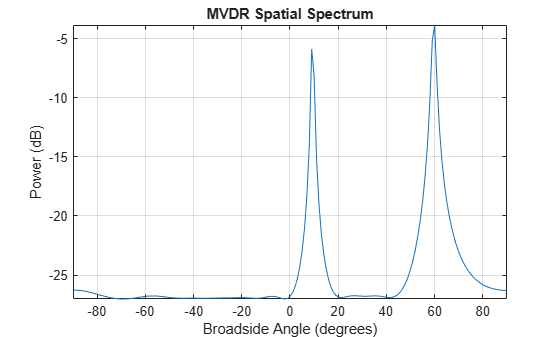# phased.MVDREstimator

MVDR (Capon) spatial spectrum estimator for ULA

## Description

The `MVDREstimator` object computes a minimum variance distortionless response (MVDR) spatial spectrum estimate for a uniform linear array. This DOA estimator is also referred to as a Capon DOA estimator.

To estimate the spatial spectrum:

1. Define and set up your MVDR spatial spectrum estimator. See Construction.

2. Call `step` to estimate the spatial spectrum according to the properties of `phased.MVDREstimator`. The behavior of `step` is specific to each object in the toolbox.

Note

Starting in R2016b, instead of using the `step` method to perform the operation defined by the System object™, you can call the object with arguments, as if it were a function. For example, ```y = step(obj,x)``` and `y = obj(x)` perform equivalent operations.

## Construction

`H = phased.MVDREstimator` creates an MVDR spatial spectrum estimator System object, `H`. The object estimates the incoming signal's spatial spectrum using a narrowband MVDR beamformer for a uniform linear array (ULA).

`H = phased.MVDREstimator(Name,Value)` creates object, `H`, with each specified property Name set to the specified Value. You can specify additional name-value pair arguments in any order as (`Name1`,`Value1`,...,`NameN`,`ValueN`).

## Properties

 `SensorArray` Handle to sensor array Specify the sensor array as a handle. The sensor array must be a `phased.ULA` object. Default: `phased.ULA` with default property values `PropagationSpeed` Signal propagation speed Specify the propagation speed of the signal, in meters per second, as a positive scalar. Default: Speed of light `OperatingFrequency` System operating frequency Specify the operating frequency of the system in hertz as a positive scalar. The default value corresponds to 300 MHz. Default: `3e8` `NumPhaseShifterBits` Number of phase shifter quantization bits The number of bits used to quantize the phase shift component of beamformer or steering vector weights. Specify the number of bits as a non-negative integer. A value of zero indicates that no quantization is performed. Default: `0` `ForwardBackwardAveraging` Perform forward-backward averaging Set this property to `true` to use forward-backward averaging to estimate the covariance matrix for sensor arrays with conjugate symmetric array manifold. Default: `false` `SpatialSmoothing` Spatial smoothing Specify the number of averaging used by spatial smoothing to estimate the covariance matrix as a nonnegative integer. Each additional smoothing handles one extra coherent source, but reduces the effective number of element by 1. The maximum value of this property is M–2, where M is the number of sensors. Default: `0`, indicating no spatial smoothing `ScanAngles` Scan angles Specify the scan angles (in degrees) as a real vector. The angles are broadside angles and must be between –90 and 90, inclusive. You must specify the angles in ascending order. Default: `-90:90` `DOAOutputPort` Enable DOA output To obtain the signal's direction of arrival (DOA), set this property to `true` and use the corresponding output argument when invoking `step`. If you do not want to obtain the DOA, set this property to `false`. Default: `false` `NumSignals` Number of signals Specify the number of signals for DOA estimation as a positive scalar integer. This property applies when you set the `DOAOutputPort` property to true. Default: `1`

## Methods

 plotSpectrum Plot spatial spectrum reset Reset states of MVDR spatial spectrum estimator object step Perform spatial spectrum estimation
Common to All System Objects
`release`

Allow System object property value changes

## Examples

collapse all

First, estimate the DOAs of two signals received by a standard 10-element ULA with element spacing of 1 meter. The antenna operating frequency is 150 MHz. The actual direction of the first signal is 10° in azimuth and 20° in elevation. The direction of the second signal is 60° in azimuth and −5° in elevation. Then, plot the MVDR spatial spectrum.

Create the signals with added noise. Then, create the ULA System object™.

```fs = 8000; t = (0:1/fs:1).'; x1 = cos(2*pi*t*300); x2 = cos(2*pi*t*400); array = phased.ULA('NumElements',10,'ElementSpacing',1); array.Element.FrequencyRange = [100e6 300e6]; fc = 150.0e6; x = collectPlaneWave(array,[x1 x2],[10 20;60 -5]',fc); noise = 0.1*(randn(size(x)) + 1i*randn(size(x)));```

Construct MVDR estimator System object.

```estimator = phased.MVDREstimator('SensorArray',array,... 'OperatingFrequency',fc,'DOAOutputPort',true,'NumSignals',2);```

Estimate the DOAs.

```[y,doas] = estimator(x + noise); doas = broadside2az(sort(doas),[20 -5])```
```doas = 1×2 9.5829 60.3813 ```

Plot the spectrum.

`plotSpectrum(estimator)`## References

 Van Trees, H. Optimum Array Processing. New York: Wiley-Interscience, 2002.

## Version History

Introduced in R2011a